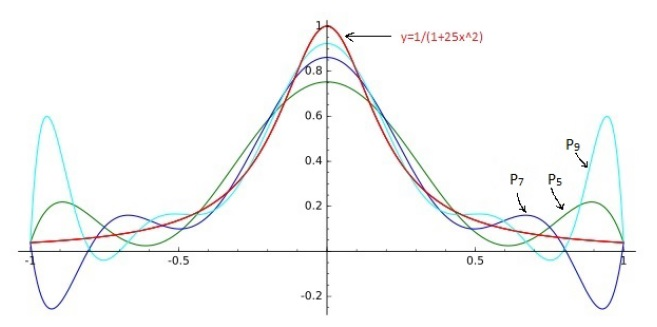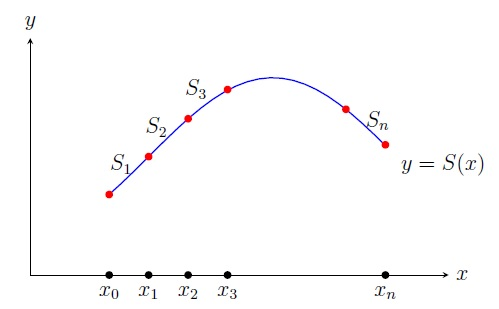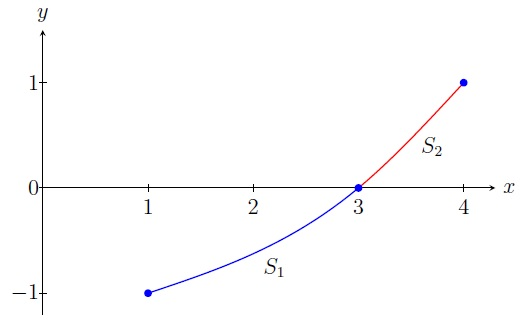Numerical Analysis Home

## Cubic Spline Interpolation

There are some problems in approximating an unknown function $$f$$ on $$[x_0,x_n]$$ by a single polynomial $$P_n$$ using $$n+1$$ points $$(x_0,y_0),\;(x_1,y_1),\ldots,(x_n,y_n)$$:
The values $$P_n(x)$$ may oscillate near the end points (Runge's phenomenon) and then the maximum error $$|f(x)-P_n(x)|\to \infty$$ as $$n\to \infty$$, i.e., the error grows for more points. For example, consider the Runge's function $$f(x)=1/(1+25x^2)$$ on $$[-1,1]$$.To avoid these problems, we use a piecewise interpolating polynomial $$S$$ on the intervals $$[x_0,x_1]$$, $$[x_1,x_2]$$, $$\ldots,[x_{n-1},x_n]$$. This is called piecewise polynomial interpolation. But in the piecewise linear interpolation, the piecewise linear polynomial $$S$$ is not smooth i.e., $$S'(x)$$ is not continuous at $$x_0,x_1,\ldots,x_n$$. If $$S$$ is piecewise quadratic, then we get the smoothness but it does not work when $$S'(x_0)$$ and $$S'(x_n)$$ are given. So we will study piecewise cubic interpolation.A spline $$S$$ of degree $$k$$ is a piecewise polynomial of degree at most $$k$$ such that $$S^{(k-1)}$$ is continuous. A cubic spline is a piecewise cubic with continuous first and second derivatives:

1. $$S(x)=S_i(x)$$ on $$[x_{i-1},x_i]$$ for $$i=1,\ldots,n$$,

2. $$S_i(x_i)=y_i=S_{i+1}(x_i)$$ for $$i=1,\ldots,n-1$$,
$$S_1(x_0)=y_0$$, and $$S_n(x_n)=y_n$$,

3. $$S_i'(x_i)=S_{i+1}'(x_i)$$ for $$i=1,\ldots,n-1$$,

4. $$S_i''(x_i)=S_{i+1}''(x_i)$$ for $$i=1,\ldots,n-1$$.

A cubic spline $$S$$ is called natural if $$S''(x_0)=S''(x_n)=0$$ and clamped if $$S'(x_0)$$ and $$S'(x_n)$$ are provided.

Example. Approximate $$f(2)$$ by constructing a natural cubic spline $$S$$ from the following data: $\begin{array}{|r|r|} \hline x & f(x)\\ \hline 1 & -1\\ \hline 3 & 0\\ \hline 4 & 1\\ \hline \end{array}$
Solution. We define $$S$$ piecewise on $$[1,4]$$ as follows: $S(x)= \begin{cases} S_1(x)=a_1+b_1(x-1)+c_1(x-1)^2+d_1(x-1)^3 & \text{if } x\in [1,3]\\ S_2(x)=a_2+b_2(x-3)+c_2(x-3)^2+d_2(x-3)^3 & \text{if } x\in [3,4]. \end{cases}$ Using the conditions of cubic spline together with the natural boundary conditions, we get a system of 8 equations in 8 variables: \begin{aligned} & S_1(1)=-1 &&\implies a_1=-1\\ & S_1(3)=0 &&\implies a_1+2b_1+4c_1+8d_1=0\\ & S_2(3)=0 &&\implies a_2=0\\ & S_2(4)=1 &&\implies a_2+b_2+c_2+d_2=1\\ & S_1'(3)=S_2'(3) &&\implies b_1+4c_1+12d_1=b_2\\ & S_1''(3)=S_2''(3) &&\implies 2c_1+12d_1=2c_2\\ & S_1''(1)=0 &&\implies 2c_1=0\\ & S_2''(4)=0 &&\implies 2c_2+6d_2=0 \end{aligned} Solving (using linear algebra) we get the unique solution $(a_1,b_1,c_1,d_1,a_2,b_2,c_2,d_2)=\left(-1,\frac{1}{3},0,\frac{1}{24},0,\frac{5}{6},\frac{1}{4},-\frac{1}{12}\right).$ Thus $S(x)= \begin{cases} -1+ \frac{1}{3}(x-1)+\frac{1}{24}(x-1)^3 & \text{if } x\in [1,3]\\ \frac{5}{6}(x-3)+\frac{1}{4}(x-3)^2-\frac{1}{12}(x-3)^3 & \text{if } x\in [3,4]. \end{cases}$ Thus $$f(2)\approx S(2)=-\frac{15}{24}$$.Note in the preceding example that we got a unique solution and hence a unique natural cubic spline. But we did not just get lucky because this is the case always:

Theorem. There is a unique natural and clamped cubic spline passing through $$n+1$$ points $$(x_0,y_0),\;(x_1,y_1),\ldots,(x_n,y_n),\; n\geq 2$$.

Proof. (Sketch) We have total $$4n$$ unknown coefficients $$a_i,b_i,c_i,d_i,\;i=1,\ldots,n$$ in $$n$$ cubics $$S_1,\ldots,S_n$$. Using $$4n-2$$ conditions of cubic spline together with 2 natural or clamped boundary conditions, we get a system of $$4n$$ equations in $$4n$$ variables. Using algebraic substitutions and linear algebra (steps skipped), we get a unique solution. ◼

Note that a clamped cubic spine usually gives better approximation than that of a natural cubic spine near the endpoints of $$[x_0,x_n]$$.

Last edited